# average current mode control of switching power supplies

#### anhnha

Joined Apr 19, 2012
901
I am reading about average current mode control of switching power supply from this application note.
In the example 1 of the app note, the author said "Near fs, the amplifier gain is flat. The overall current loop has only one active pole (from the inductor)." Can anyone help me to understand it intuitively and quantitatively?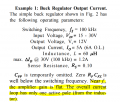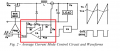For the current compensation in red box, I derived the transfer function as below: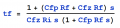However, I don't know how to arrive at the conclusion given above.

#### MrAl

Joined Jun 17, 2014
9,355
I am reading about average current mode control of switching power supply from this application note.
In the example 1 of the app note, the author said "Near fs, the amplifier gain is flat. The overall current loop has only one active pole (from the inductor)." Can anyone help me to understand it intuitively and quantitatively?

View attachment 147597

View attachment 147598

For the current compensation in red box, I derived the transfer function as below:

View attachment 147599

However, I don't know how to arrive at the conclusion given above.
Hi,

First, they are saying that Cfp is temporarily omitted.
Second, what is that block "VA" doing for us?

It looks like a somewhat simple integrator question. That is, how an integrator with feedback series resistance works given a certain frequency range, because of the factor Rf/Ri, which forms a simple gain independent of frequency.
So what is being said seems to be that Rf/Ri dominates near a certain frequency, so that puts a limit on the range of the size of Cfz.

To test, just make 1/RC much lower than the switching frequency, then look at the response over a small range of frequencies near the switching frequency. To get more precise, make the frequency range Fs-k and Fs+k, make the RC combo something, then look for the 3db points which may be one at -3db and one at +3db, but solve for k. I would think the frequency range should be at least plus and minus 10 percent.

Last edited:

#### anhnha

Joined Apr 19, 2012
901
Hi MrAl,

With Cfp is omitted, the transfer function of the current amplifier is simplified but it still has a pole at origin. So the overall should at least have one pole at origin.
Also, how to model to derive the current loop gain? I would like to calculate current loop gain and see poles, zero it has.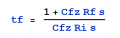For VA, I don't see it mentioned anywhere in the article. However, I believe it is a voltage amplifier to compensate for the voltage loop.
It is similar to this figure from Maxim app note DC-DC Controllers Use Average-Current-Mode Control: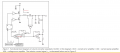#### MrAl

Joined Jun 17, 2014
9,355
Hi MrAl,

With Cfp is omitted, the transfer function of the current amplifier is simplified but it still has a pole at origin. So the overall should at least have one pole at origin.
Also, how to model to derive the current loop gain? I would like to calculate current loop gain and see poles, zero it has.

View attachment 147610

For VA, I don't see it mentioned anywhere in the article. However, I believe it is a voltage amplifier to compensate for the voltage loop.
It is similar to this figure from Maxim app note DC-DC Controllers Use Average-Current-Mode Control:

View attachment 147611

Hello again,

Expand that into a better form and you might see this right away. The main idea simplified is that the cap has to look like a short circuit for the frequencies around the switching frequency, and that makes the amp look like a simple gain block with no frequency dependence (near the Fs).
Also, the gain should be negative.

The switcher part is often modeled as a simple gain, assuming an average response. This means for example if we have a 50 percent duty cycle then we have a gain of 1/2, and 33.3333 percent duty cycle then we have a gain of 1/3, etc. Max is 100 percent which is a gain of 1. Min often does not go to zero, but for this you can probably assume that it does go to zero for zero percent duty cycle.

#### anhnha

Joined Apr 19, 2012
901
Hi MrAl,

By expanding it, I got this: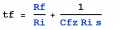I think the assumption here is that at switching frequency fs the second terms is much smaller than the first time so we can ignore it.
However, I am still confused about the number of pole that the article said. No matter how we expand it, we always have one origin pole.
So the current loop gain should have at least two poles, one at origin and one active pole created by the inductor.
How can we consider it as one pole system?

The switcher part is often modeled as a simple gain, assuming an average response. This means for example if we have a 50 percent duty cycle then we have a gain of 1/2, and 33.3333 percent duty cycle then we have a gain of 1/3, etc. Max is 100 percent which is a gain of 1. Min often does not go to zero, but for this you can probably assume that it does go to zero for zero percent duty cycle.
I think I see the idea. How can we model it including inductor L so we can calculate exactly the loop gain and locate where the pole, zero is?

#### anhnha

Joined Apr 19, 2012
901

#### MrAl

Joined Jun 17, 2014
9,355
Hi MrAl,

By expanding it, I got this:

View attachment 147613

I think the assumption here is that at switching frequency fs the second terms is much smaller than the first time so we can ignore it.
However, I am still confused about the number of pole that the article said. No matter how we expand it, we always have one origin pole.
So the current loop gain should have at least two poles, one at origin and one active pole created by the inductor.
How can we consider it as one pole system?

I think I see the idea. How can we model it including inductor L so we can calculate exactly the loop gain and locate where the pole, zero is?
Hello again,

It is not unusual to reduce the order of a system to a lower order so that we can either understand it better or do a simpler analysis and thus gain usable data as well as insight into the working of the circuit. For this i guess you would have to do the analysis to find out.
This thing about the current loop is that we are sensing current in the inductor and that is significant because controlling that leads to a very fast response and i think it's considered nearly first order. We can find out more though.

Block the system as follows and see if you can do it yourself first...
1. Amplifier block as you have done already.
2. Gain block for the PWM modulator, a simple gain of 0 to 1 depending on the output of the amplifier.
3. #2 puts a linear signal into the inductor, no longer pulses, so it's an inductor driven by essentially a zero output impedance amplifier.
4. The output side of the inductor has to go to the cap and load as usual.

So it is like:
FBAmp--->PWMgain--->inductor--->OutputSection--->(FBAmp)

In the above (FBAmp) is the same as FBAmp it's the point where the output current goes back into the Amp.

It is probably simpler to view this system in state vector form.
The current in the cap is related to a derivative, but the current in the inductor is just a state variable, so it's basically state variable feedback.

See what you can come up with, and we'll go from there if you like. Keep in mind these are all just simple gain blocks in the averaged model. The inductor of course stays an inductor, but it is driven now by a linear voltage source provided by the PWM modulator averaged model.

Maybe this is a lot to take in all at once, but see what you can make of it.

#### anhnha

Joined Apr 19, 2012
901
Hi MrAl,

1. Amplifier block as you have done already.
2. Gain block for the PWM modulator, a simple gain of 0 to 1 depending on the output of the amplifier.
I see that this block is model as a gain of 1/Vs where Vs is the peak to peak voltage of the ramp signal.
3. #2 puts a linear signal into the inductor, no longer pulses, so it's an inductor driven by essentially a zero output impedance amplifier.
Is it modeled like this? And then what drive inductor here? I think it should be duty cycle which is the output of PWM block. So I am not sure what gain should I calculate in the block #3.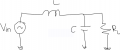For state vector, I don't quite understand what are you saying. Could you elaborate?

#### MrAl

Joined Jun 17, 2014
9,355
Hi MrAl,

I see that this block is model as a gain of 1/Vs where Vs is the peak to peak voltage of the ramp signal.

Is it modeled like this? And then what drive inductor here? I think it should be duty cycle which is the output of PWM block. So I am not sure what gain should I calculate in the block #3.

View attachment 147631

For state vector, I don't quite understand what are you saying. Could you elaborate?
Hi again,

I just saw your previous post.

Actually this is much easier than i thought at first. We dont have to bother with averaging the cap voltage, we can just set that to (for this problem) 12 volts DC. After that, you can see that the circuit is just a feedback amp and inductor. The feedback amp can feed the inductor, and the inductor output connects to the DC source of +12v.
The scale of the output of the amp is determined by the Vs (i think they call it that here) which i think is 30v. That means the max output of the amp is 30v and the min is 0v. The average output is 12v because the output is 12v.

So you see what happens with a change of current on the output. The current sense senses that current, amplifies it by Rf/Ri (assuming Rs=1 ohm), then multiplies that by the PWM gain which is 0 to 1, then applies that to the inductor, and that increases the inductor voltage and thus causes a ramp in current going up to supply the output with the required increase in current (or somewhere around that amount).
Because the amp is considered non frequency sensitive that leaves only the inductor, and a single inductor would only give us a first order system.
The actual response would come out to A-A*e^(-a*t) but because we are dealing with short time periods it could be thought of as just:
v=L*di/dt
so:
di=v*dt/L

so we can think of it as a ramp up for small values of dt.

Is this starting to make sense now?

I think the output of the amp should increase as the output current demand increases but we could check into that to make sure it is not the opposite. I cant see how lowering the drive signal could help the output if the output current increased though.
It looks like they set it up that way because of the waveshape they show for the PWM ramp, but sometimes they dont show that right.

Last edited:

#### anhnha

Joined Apr 19, 2012
901
Hi MrAl,

The scale of the output of the amp is determined by the Vs (i think they call it that here) which i think is 30v. That means the max output of the amp is 30v and the min is 0v. The average output is 12v because the output is 12v.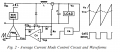In this figure, Vs is peak-to-peak voltage of the ramp signal. It is 5V in this example.
Vin ranges from 15 to 30V and Vout is 15V.
So the output of the amplifier should be between 0 and 5V.

So you see what happens with a change of current on the output. The current sense senses that current, amplifies it by Rf/Ri (assuming Rs=1 ohm), then multiplies that by the PWM gain which is 0 to 1, then applies that to the inductor, and that increases the inductor voltage and thus causes a ramp in current going up to supply the output with the required increase in current (or somewhere around that amount).
Does the output current increase or decrease in that assumption? If output load current increases then because the inverting amplifier with gain -Rf/Ri the output of the amplifier Vca decreases. The results in duty cycle decreases.
It doesn't seem to make sense to me.

#### MrAl

Joined Jun 17, 2014
9,355
Hi MrAl,

View attachment 147691

In this figure, Vs is peak-to-peak voltage of the ramp signal. It is 5V in this example.
Vin ranges from 15 to 30V and Vout is 15V.
So the output of the amplifier should be between 0 and 5V.

Does the output current increase or decrease in that assumption? If output load current increases then because the inverting amplifier with gain -Rf/Ri the output of the amplifier Vca decreases. The results in duty cycle decreases.
It doesn't seem to make sense to me.

Hello again,

It must be that when the output current increases the duty cycle increases. That makes sense.

Here is a circuit that shows what we are looking at. Note the switching part has been removed and replaced with an amplifier with a gain of -Vin/5. We assume that Vin is stable over some medium length time duration so the gain is ocnstant. The gain is set like that because you wanted to use 5v max output of the CA amplifier.
A positive change in output current dI would lead to a negative change in output voltage dVo over a very short time, then the duty cycle would increase and make up for the changes.

Can you do the analysis now of the current sense loop?

.

#### anhnha

Joined Apr 19, 2012
901
Hi MrAl,

I think I can calculate the loop gain now but I have a few questions which I don't quite understand.
You have a triangle block with the gain of -Vin/5.

Why the gain is negative and why Vin is involved here?

#### MrAl

Joined Jun 17, 2014
9,355
Hi MrAl,

I think I can calculate the loop gain now but I have a few questions which I don't quite understand.
You have a triangle block with the gain of -Vin/5.

Why the gain is negative and why Vin is involved here?
Hi,

Why the gain is negative...
The waveform shown on the original drawing seems to indicate that as the current increases the output of the CA amp goes down, and that creates a higher duty cycle. But even more striking is that the voltage loop must have negative feedback to regulate the voltage.
[LATER NOTE:
A problem is that they dont show the voltage amp details. If we make that a negative feedback amp then the gain shown as -Vin/5 would have to be +Vin/5. That would make sense as then the current feedback would not be positive. We'll have to look at this more closely. It's a shame they did not show the details of the voltage amp VA.]

Vin is involved because that is the mechanism we can use to get the averaged Vout=Vin with the equivalent 100 percent duty cycle.

Really all we are after is a linear version of the original buck circuit. It should work the same as the original except no switching actions.

Last edited:

#### anhnha

Joined Apr 19, 2012
901
Hi MrAl,

Why the gain is negative...
The waveform shown on the original drawing seems to indicate that as the current increases the output of the CA amp goes down, and that creates a higher duty cycle. But even more striking is that the voltage loop must have negative feedback to regulate the voltage.
I see it now. The input switch is a pmos transistor so output of CA down means it turns on longer.

Vin is involved because that is the mechanism we can use to get the averaged Vout=Vin with the equivalent 100 percent duty cycle.

Really all we are after is a linear version of the original buck circuit. It should work the same as the original except no switching actions.
I don't really understand this. I see that usually when calculating duty cycle to output voltage or inductor current, input voltage is set to zero.

However, come back to current loop gain Ti:Ti = -Vin/5 * 1/(s*L) *Rs* G where G is the gain of CA amp.

#### MrAl

Joined Jun 17, 2014
9,355
Hi MrAl,

I see it now. The input switch is a pmos transistor so output of CA down means it turns on longer.

I don't really understand this. I see that usually when calculating duty cycle to output voltage or inductor current, input voltage is set to zero.

However, come back to current loop gain Ti:Ti = -Vin/5 * 1/(s*L) *Rs* G where G is the gain of CA amp.
Hello,

When i do the analysis i just allow the output of CA to go to anything it wants to be, because i assume that the feedback will limit the output. But because you wanted the output of CA to be a max of 5 volts, and because we want a max Vout of the circuit to be 30 volts with 30 volts input, we can use Vin to limit the output. That's not mandatory though. If you feel more comfortable with getting rid of Vin, then just allow the output of CA to go to whatever it needs to be in order to bring the circuit to steady state, then you dont even need the gain stage -Vin/5 at all.
BTW it looks like it should be +Vin/5 now because the missing VA amp is probably an inverting op amp integrator stage. If you eliminate that stage and let the output of CA go to anything it wants to be, then you can just short that stage out or make it a gain of +1.

I find that when i do the above, i get 10v out when the reference for the VA amp is set to 10v, and with no current feedback i get a long transition when the output current is increased, but with current feedback i get a shorter transition (transition between when steady state is lost to when steady state is regained). I threw some values in there to get going. L=1mH for example.

I am thinking you may want to go to a numerical analysis for this circuit because there are at least two storage elements that have to be tracked during a full blown analysis and that gets a little more sticky then most people want to get involved with in the age of circuit simulators. Also of course a simulator helps a lot too.

The numerical analysis isnt that difficult but you should have access to some programming language so you can program in the steps to solve it. That way you can do many points in time in the blink of an eyeThe programming is very simple, mostly math steps like adding and subtracting and multiplication and division.

Last edited:

#### anhnha

Joined Apr 19, 2012
901
Hi MrAl,

Let's use Vin/5 and assume that VA is an inverting amplifier. That is maybe easier to get started.
Also numerical and programming is fine too. However, I am not sure how to start.
Could you you write down steps to do that?

On page 3 of the article:

Average current mode control has a very similar problem, but a better solution. The oscillator ramp effectively provides a great amount of slope compensation. One criterion applies in a single pole system: The amplified inductor current downslope at one input of the PWM comparator must not exceed the oscillator ramp slope at the other comparator input. This criterion puts an upper limit on the current amplifier gain at the switching frequency, indirectly establishing the maximum current loop gain crossover frequency, fc. It is the first thing that needs to be considered in optimizing the average current mode control loop.

Why do we only care about downslope of inductor current not up slope?

Last edited:

#### MrAl

Joined Jun 17, 2014
9,355
Hi MrAl,

Let's use Vin/5 and assume that VA is an inverting amplifier. That is maybe easier to get started.
Also numerical and programming is fine too. However, I am not sure how to start.
Could you you write down steps to do that?

On page 3 of the article:

Average current mode control has a very similar problem, but a better solution. The oscillator ramp effectively provides a great amount of slope compensation. One criterion applies in a single pole system: The amplified inductor current downslope at one input of the PWM comparator must not exceed the oscillator ramp slope at the other comparator input. This criterion puts an upper limit on the current amplifier gain at the switching frequency, indirectly establishing the maximum current loop gain crossover frequency, fc. It is the first thing that needs to be considered in optimizing the average current mode control loop.

Why do we only care about downslope of inductor current not up slope?
Hello again,

Well i would have to look at the circuit you are talking about here with this new question, but what i was hoping to do was to get you to realize that there could be literally thousands of questions when it comes to these circuits and the best way to get familiar with them is to sit down, roll your sleeves up, and do some hard analysis. If you dont feel up to it, you can use a simulator. Better yet though, do an analysis and then compare your results to the simulator so you can be sure you have the right equations and thus the same results.

We would probably do a simpler circuit first so you can get the hang of using the numerical solution methods. This quickly illustrates the technique. As i said, this requires a little programming but if you did even a little programming in BASIC you can easily handle this. In fact, you probably wont believe how simple it really is once you get going.

If we start with a regular resistor and capacitor circuit (as in another thread here on AAC) and driven by a step input, and we want the time solution to the capacitor voltage, we can write an equation. Note this is just a resistor R1 in series with a cap C1 and driven by a voltage step source E1. The equation could start:
(E1-v)/R1=C*dv/dt {v is the cap voltage}

and note all we did here was find the voltage across the resistor R1 and divide by R1 to get the current, then equate that to the capacitor current (on the right).

We really want the solution to the cap voltage but we have to solve the differential equation. There are of course various ways to do this but we want to do it numerically this time. Since we need to solve for the derivative as part of the solution, we can put that on the left side:
(E1-v)/R1=C*dv/dt
C*dv/dt=(E1-v)/R1

then solve for the derivative:
dv/dt=(E1-v)/(R1*C1)

and expanding the right side:
dv/dt=E1/(R1*C1)-v/(R1*C1)

Now we have the equation in ODE form. The deriv is on the left and everything else on the right.

The first solution method we can use is sometimes called "Euler's Method" but it's really just a first order Taylor Method (it helps to recognize this later with more complicated circuits). It is derived from a Taylor Series.

Anyway, the solution could not be simpler as we view each solution point as a pair of discrete values (v,t):
v[n+1]=v[n]+h*dv/dt
t[n+1]=t[n]+h

and here v[n] is the previous value of v and dv/dt is calculated directly from the derivative and h is the time increment.
t[n+1] is just the next time value and t[n] the previous where we had solution v[n].

In simpler notation, that looks like this:
v=v+h*dv/dt
t=t+h

All we do is assume some starting value for v, like zero (0), then start calculating the two equations over and over again.
An interesting view of the derivative:
dv/dt=E1/(R1*C1)-v/(R1*C1)

is that we are going to be using the right side over and over again exactly as it is written. This leads to a new variable we acn call simply DV:
DV=E1/(R1*C1)-v/(R1*C1)

and this is a line of code in say BASIC for example.
With each calculation we get a new value of DV and a new value of time:
t=t+h

and a new value for the voltage v:
v=v+h*DV

So we just keep calculating them over and over again, and obtain a new value of v for each new value of time t, and we can plot the results.

Ok, so an example would be R1=1, C1=1, E1=1, h=0.1 (trying to keep this simple).
From:
DV=E1/(R1*C1)-v/(R1*C1)

that means we get:
DV=1-v

ahd the solution equations:
v=v+h*DV
t=t+h

turn into:
v=v+0.1*(1-v)
t=t+0.1

We could simplify v a litte more, but lets do some raw math next.
Starting with v=0 we have first:
v=0+0.1*(1-0)=0.1
t=t+0.1=0.1

So our first point is (0.1, 0.1).
Now repeat:
v=v+0.1*(1-v)
t=t+0.1

so:
v=0.1+0.1*(1-0.1)=0.1+0.1-0.01=0.19
t=0.1+0.1=0.2

and the next time step:
v=0.19+0.1*(1-0.19)=0.271
t=0.2+0.1=0.3

If we do this until t=1 we get:
v=0.6513215599

and the more exact solution is:
v=0.63212055883

so you can see we got the approximate solution using the numerical method.
If we start over and use h=0.01, we get v=0.633968, and if we use h=0.001 we get v=0.632305
so we can see that as we make h smaller we get more accurate results, although there is a limit to how low we can go before it gets worse.

There are also other methods like Heun's Method that attempts to get a better result with larger h. There are a lot of even better methods too usually classified by the order of accuracy. The one here was first order, but they can go up much higher.

#### anhnha

Joined Apr 19, 2012
901
Hi MrAl,

I think you gave an example of series RC circuit just to illustrate how to solve it numerically. So for the original circuit what equation needed to solve by using this method?
I am not sure how you apply this method for the buck converter above.

#### MrAl

Joined Jun 17, 2014
9,355
Hi MrAl,

I think you gave an example of series RC circuit just to illustrate how to solve it numerically. So for the original circuit what equation needed to solve by using this method?
I am not sure how you apply this method for the buck converter above.
Hi again,

Ok let's do a buck next then. I havent actually done this in a long time now so it will be interesting for me too.
Give me a little while to prepare something...
I'll post again so you get another alert.

•Merchant and anhnha

#### MrAl

Joined Jun 17, 2014
9,355
Hi,

Here is a quick buck analysis. A couple notes here.

The program used was an embedded form of a free language and it's quite simple so i used that.

The buck circuit is shown in 'text' because i happened to have it already drawn for another problem in another thread.
The analysis was for a single pulse, that starts from t=0 and ends at t=0.1 and then stays at zero for another 0.1 seconds so the total analysis goes from 0 to 0.2 seconds. The pulse amplitude is E1 and was made equal to 1 for this quick test.

This is a quick example of how using this technique can solve networks. The solution method was first order so a small time increment (h) was used.

For best viewing you should probably download and view in a good paint program. IF it is not clear enough i'll upload a text version instead.
[Note is was not that clear in the browser so i uploaded a text file too which can be viewed in any text editor using a non proportional font]

#### Attachments

• 45.3 KB Views: 6
• 1.4 KB Views: 10